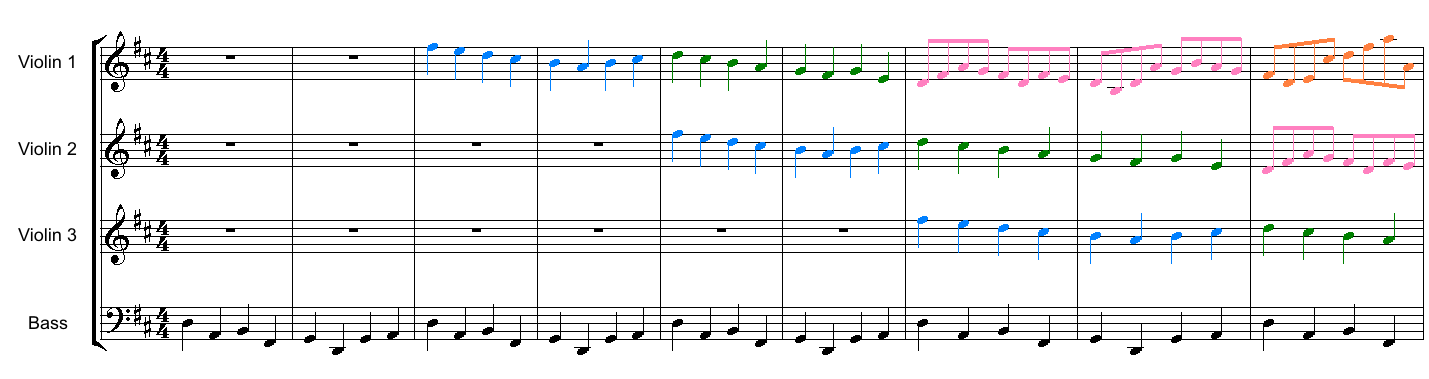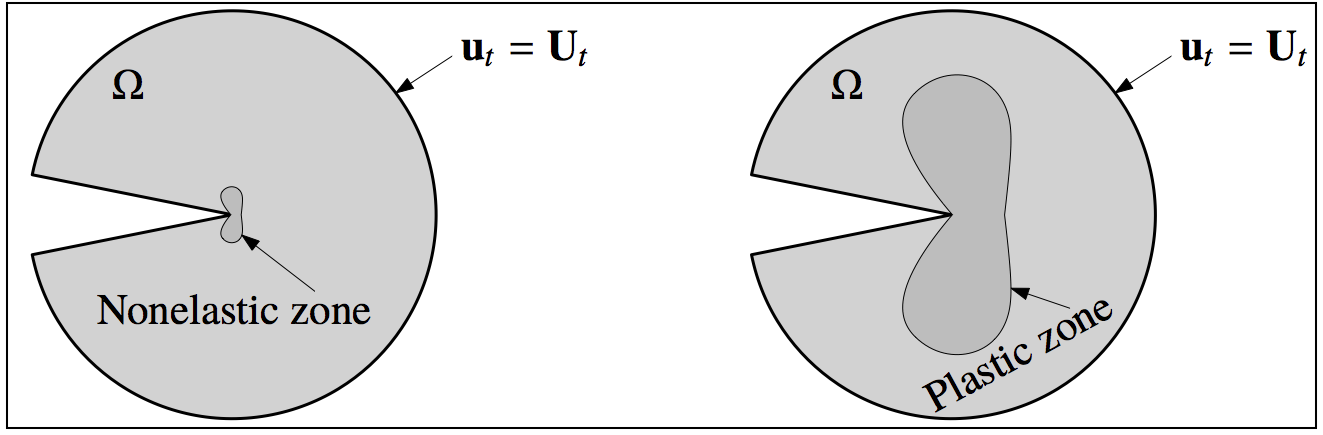# 从卡农到递归可枚举

18 分钟阅读

• 音乐里面可以说是主旋律和伴奏之间的关系；
• 绘画里面就是图形和衬底之间的关系；
• 数学里面可以有一个集合及其补集之间的关系。

### 卡农

「卡农」这个词到不是第一次见到，不过以前见到的时候通常指的是下面这首曲子：D 大调卡农与吉格 (Pachelbel’s Canon)。下面你可以尝试听听 Jeffrey C. Hall 在 Wikimedia Commons 上通过 CC BY-SA 3.0 协议共享的电子合成版本。$f_2(t)=f_1(t-T)\,,\qquad f_3(t)=f_2(t-T)=f_1(t-2T)$

### Escher

1. 用画笔画到的地方作为图形；
2. 没有被画到的部分作为衬底。### 递归可枚举

$\overline{S}=\{\,x\in\mathbb{N}\,|\,x\notin S\,\}$

$n=P(i)+P(j)\,,\qquad 1\leq i\leq j$

$$j=1$$ $$j=2$$ $$j=3$$ $$j=4$$
$$i=1$$ $$k=1$$ $$k=2$$ $$k=4$$ $$k=7$$
$$i=2$$   $$k=3$$ $$k=5$$ $$k=8$$
$$i=3$$     $$k=6$$ $$k=9$$
$$i=4$$       $$k=10$$

# 计算能由两个素数的和所表示的自然数集合 S
k = 1  # 处理 k=1 的情况
S = set()  # S 一开始是空集
while True:  # 一直循环
i, j = rho(k)  # 找到对应 k 的 (i,j)
S.add( P(i) + P(j) )  # 计算 P(i)+P(j) 将结果放进 S
k = k + 1  # 接下来处理 k+1 的情况 循环


1. 先看看上面表格第一行，即对应 $$i=1$$ 所产生的数。由于第一个素数 $$P(1)=2$$ 是唯一的偶数素数，所以除了 $$P(1)+P(1)=4$$ 是偶数之外，当 $$j>i$$ 的时候其他 $$P(1)+P(j)=2+P(j)$$ 都是奇数（偶数加奇数是奇数）。这样一来第一行的数很好判断，如果是偶数那么只能是 4，如果是奇数，减去 2 必须是个素数；
2. 然后看看其他情况下所产生的数。由于排除了 $$P(1)=2$$ 的情况，所以这里由 $$2\leq i\leq j$$ 产生的 $$P(i)+P(j)$$ 肯定都是偶数（因为奇数加奇数是偶数）。问题是我们不知道到底哪些偶数能被两个素数相加获得……除非……我们证明了哥德巴赫猜想

1. 所以，在上面猜想真的成立的情况下，那么 $$S$$ 就是所有大于 2 的偶数以及所有形如 $$2+p,\,p\in\mathbb{P}$$ 的数所组成的集合。其补集很好获得，也很容易用计算机程序生成。
2. 但由于目前这个猜想还没有证明，我们不知道是不是存在哪些偶数，它们不能被两个素数相加所获得。由于无法理解 $$S$$ 在自然数集合中的具体「刻画」，所以其补集也无法用程序生成。在这种情况下我们就说 $$S$$ 是一个非递归的递归可枚举集合。它「递归可枚举」，是因为存在一个算法生成它，但由于我们对它理解（还）不透，它的补集不能被生成，所以它「非递归」。别问我这个学力学的为什么计算理论这么定义这两个形容词……

### 同构的建立

“伴奏” “衬底” 补集 $$S$$ （半）主动 传递同等重要的信息

1. 今天看完了最后一个对话，结果发现最后的最后即又轮回开始……

2. 数学事实上有很多公理体系，不过这些体系之间大致也应该是「同构的」……